# 4) What element is undergoing oxidation (if any) in the following reaction? Zn(s) + 2 AgNO3(aq)...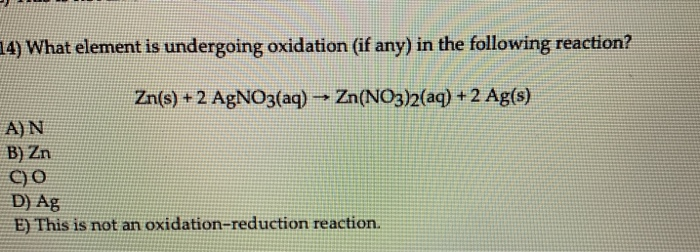4) What element is undergoing oxidation (if any) in the following reaction? Zn(s) + 2 AgNO3(aq) → Zn(NO3)2(aq) + 2 Ag(s) A) N B) Zn C) o D Ag E) This is not an oxidation-reduction reaction.

Oxidation state of Zn changes from 0 to +2

So, Zn is being oxidised.

Oxidation state of Ag is changing from +1 to 0

So, Ag is being reduced

#### Earn Coin

Coins can be redeemed for fabulous gifts.

Similar Homework Help Questions
• ### 12) Determine the oxidation state of P in phosphate, PO33- A) +3 B) +6 C) +2...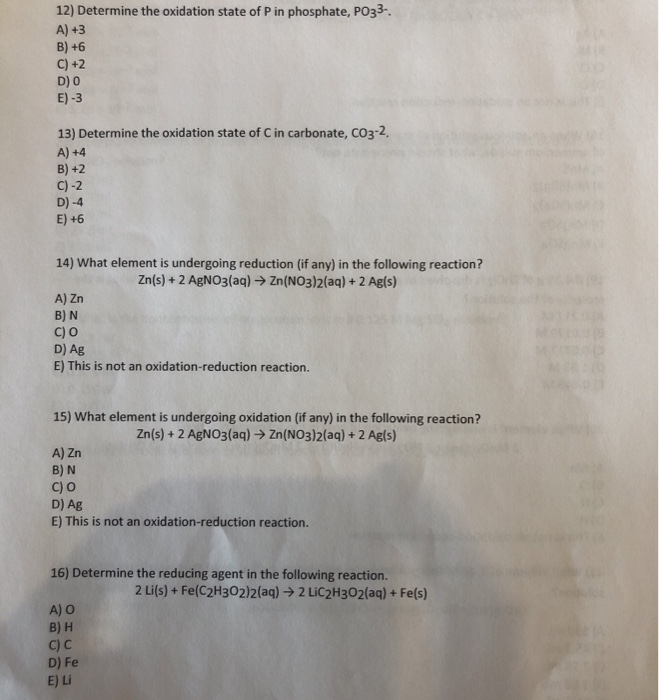12) Determine the oxidation state of P in phosphate, PO33- A) +3 B) +6 C) +2 D) O E) -3 13) Determine the oxidation state of Cin carbonate, CO3-2, A) +4 B) +2 C)-2 D) -4 E) +6 14) What element is undergoing reduction (if any) in the following reaction? Zn(s) + 2 AgNO3(aq) → Zn(NO3)2(aq) + 2 Ag(s) A) Zn B) N c) O D) Ag E) This is not an oxidation-reduction reaction. 15) What element is undergoing oxidation...

• ### Give the net ionie eauation for the reaction (if any) that occurs when aqueom ACato ad...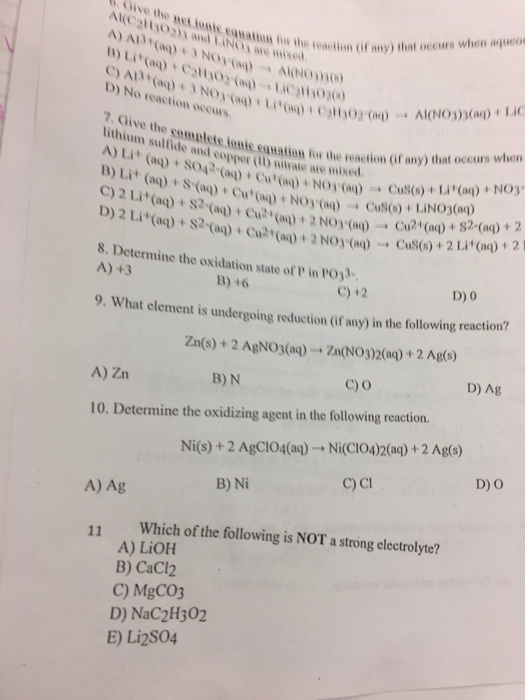Give the net ionie eauation for the reaction (if any) that occurs when aqueom ACato ad LINOS e ed (w).toN e)cv C) A(aq)+ 3NO ()Li)+ Cgtt02 (a) B) Li(aq)+ Cy0, (aq)--14C)lHi0 AlNO30 D) No reaction occurs AI(NO3)3(aq)+ LIC 7. Give the somplete ionic eauation for the reaction (if any) that occurs when lithium sulfide and copper () nitrate are mixed A) Lit (aq)+ SO42-(a)+ Cu(aq)+ NOy() B) Lit (aq)+ S(aq) + Cut(aq)+ NOy () C)2 Li (aq)+S2-(aq)+ Cu(a)+ 2 NO3...

• ### 4. Which of the following statements about the voltaic cell shown below is correct? Zn(s)[Zn** (aq)||...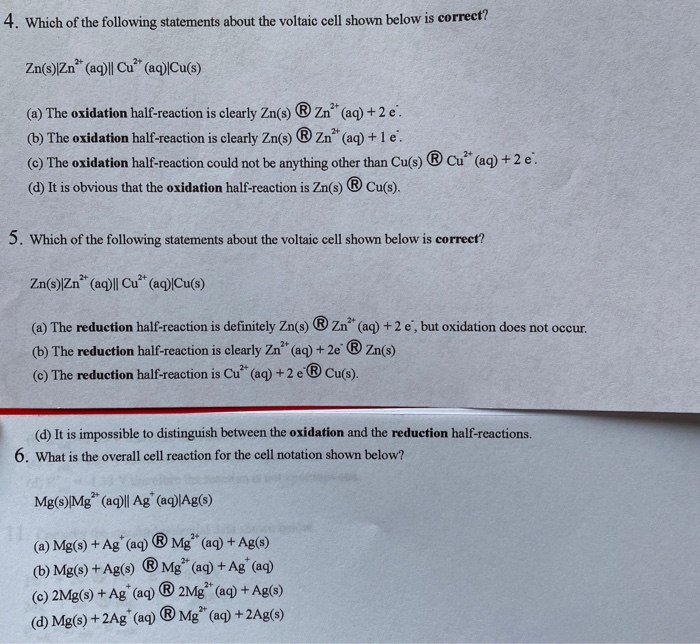4. Which of the following statements about the voltaic cell shown below is correct? Zn(s)[Zn** (aq)|| Cu** (aq)|Cu(s) (a) The oxidation half-reaction is clearly Zn(s) ® Zn** (aq) + 2 e. (b) The oxidation half-reaction is clearly Zn() Zn" (aq) +le. (C) The oxidation half-reaction could not be anything other than Cu(s) ® Cu (aq) +2 e. (d) It is obvious that the oxidation half-reaction is Zn(s) ® Cu(s). 5. Which of the following statements about the voltaic cell shown...

• ### 5. (12 points) For the following oxidation reduction reaction: Zn(s)+NO3 (aq) > Zn2*(aq) + N2(g) +...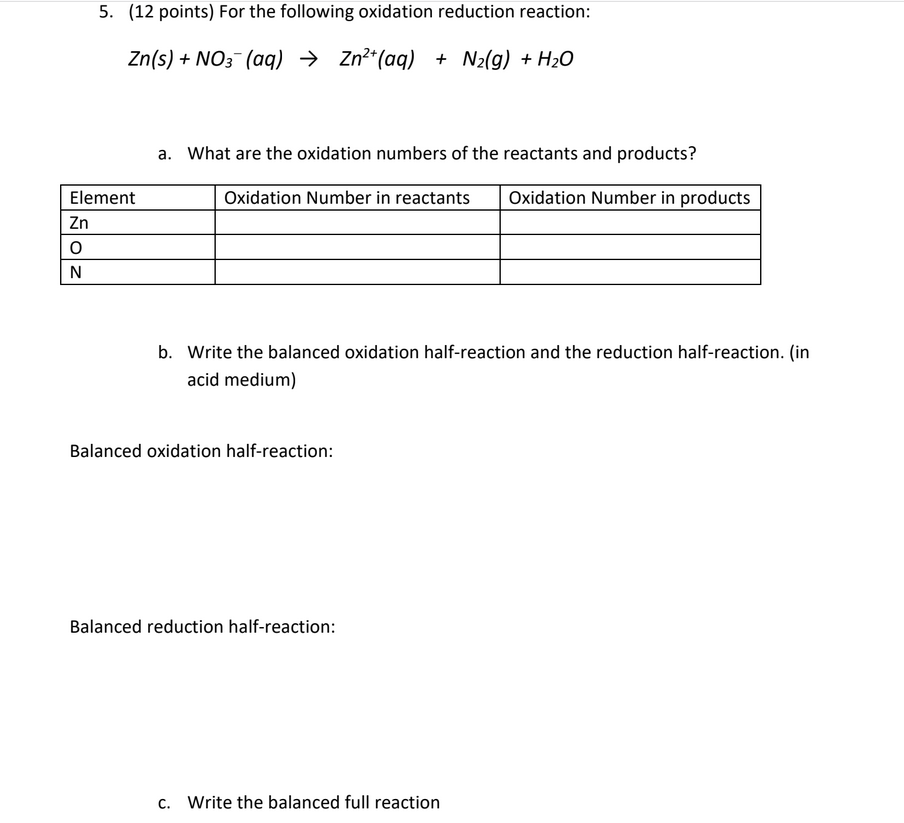5. (12 points) For the following oxidation reduction reaction: Zn(s)+NO3 (aq) > Zn2*(aq) + N2(g) + H20 a. What are the oxidation numbers of the reactants and products? Oxidation Number in products Element Oxidation Number in reactants Zn N b. Write the balanced oxidation half-reaction and the reduction half-reaction. (in acid medium) Balanced oxidation half-reaction: Balanced reduction half-reaction: Write the balanced full reaction c.

• ### 17) Determine the oxidizing agent in the following reaction. Ni(s) + 2 AgClO4(aq) → Ni(ClO4)2(aq) +...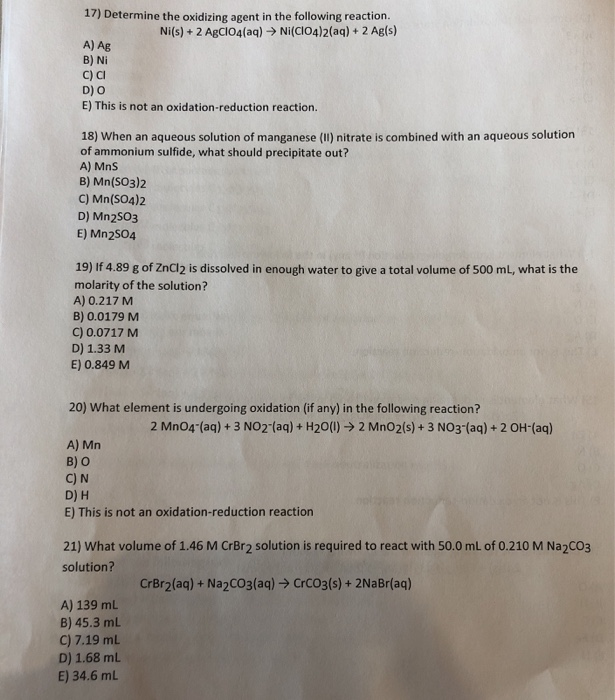17) Determine the oxidizing agent in the following reaction. Ni(s) + 2 AgClO4(aq) → Ni(ClO4)2(aq) + 2 Ag(s) A) Ag B) Ni C) CI D) O E) This is not an oxidation-reduction reaction. 18) When an aqueous solution of manganese (II) nitrate is combined with an aqueous solution of ammonium sulfide, what should precipitate out? A) Mns B) Mn(SO3)2 C) Mn(SO4)2 D) Mn 2503 E) Mn 2504 19) If 4.89 g of ZnCl2 is dissolved in enough water to give a total...

• ### Consider the following reaction. 2Ag^+(aq) + Zn(s) --> 2Ag(s) +Zn(aq) a) write the individual oxidation and...

Consider the following reaction. 2Ag^+(aq) + Zn(s) --> 2Ag(s) +Zn(aq) a) write the individual oxidation and reduction half-reactions, specifying which is the anode and which is the cathode reaction. b) calculate the cell potential for a cell containing 0.10M Ag^+ and 0.25M Zn^2+ at 298K. (E ag= 0.80 V and E zn= -0.76 V)

• ### 18) Which of the following is an acid-base reaction? A) Fe(s) + 2 AgNO3(aq) - 2...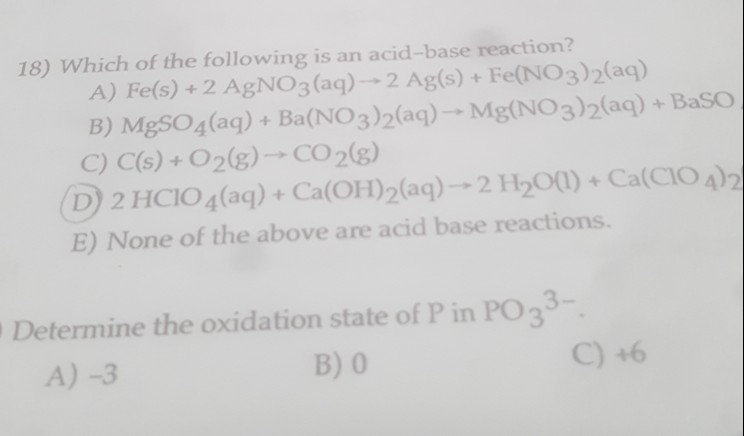18) Which of the following is an acid-base reaction? A) Fe(s) + 2 AgNO3(aq) - 2 Ag(s) + Fe(NO3)2(aq) B) MgSO4(aq) + Ba(NO3)2(aq) -- Mg(NO3)2(aq) + Baso C) C(s) + O2(g) - CO2(g) (D) 2 HCIO 4(aq) + Ca(OH)2(aq) → 2 H2O(l) + Ca(C1O4)2 E) None of the above are acid base reactions. 1 Determine the oxidation state of P in PO 33-. A-3 B) 0 C) +6

• ### What is the net ionic equation for the following reaction? 2 AgNO3(aq)   +     BaCl2(aq)   2 AgCl(s)  ...What is the net ionic equation for the following reaction? 2 AgNO3(aq)   +     BaCl2(aq)   2 AgCl(s)   +   Ba(NO3)2(aq) A. 2 Ag+(aq) +   2 Cl--(aq)    2 AgCl(s) B. Ag+(aq) +    Cl--(aq)      AgCl(s) C. Ba2+(aq)   +   2        Ba(NO3)2(aq) D. 2 Ag+(aq) + 2   + Ba2+(aq)   + 2 Cl-(aq)   2 AgCl(s) + Ba2+(aq) + 2

• ### Balance each of the following skeletal equations: (a) Mg(s)+ O2(g) (b) Zn(s)+ AgNO3(aq) Zn(NO3)2(aq) + Ag(s)...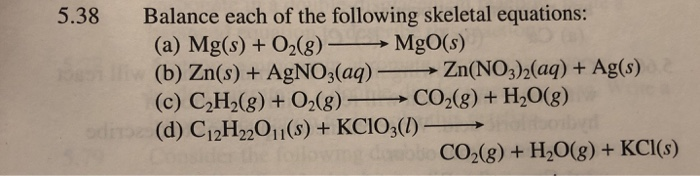Balance each of the following skeletal equations: (a) Mg(s)+ O2(g) (b) Zn(s)+ AgNO3(aq) Zn(NO3)2(aq) + Ag(s) (c) C2H2(g)+O2(g) CO2(g) + H20(g) 5.38 MgO(s) odm(d) C12H22O11(s) +KCIO3(I) Constder the foll CO2(g) +H20(g) + KCI(s)

• ### In each redox reaction identify the element undergoing oxidation and the element undergoing reduction. 4NH3(g)+5O2(g)⟶4NO(g)+6H2O(g) In...

In each redox reaction identify the element undergoing oxidation and the element undergoing reduction. 4NH3(g)+5O2(g)⟶4NO(g)+6H2O(g) In each redox reaction identify the element undergoing oxidation and the element undergoing reduction. O is oxidized, N is reduced N is oxidized, O is reduced N is oxidized, N is reduced O is oxidized, O is reduced 2NO(g)+O2(g)⟶2NO2(g) O is oxidized, N is reduced N is oxidized, O is reduced N is oxidized, N is reduced O is oxidized, O is reduced 3NO2(g)+H2O(l)→2HNO3(aq)+NO(g) O...

Free Homework App